# Learning Electronics

Learn to build electronic circuits

# Simultaneous equations for circuit analysis

### Question 1:

Solve this equation for the value of x:

 x + 5 = 8

How many exact solutions does the above equation have?

Now, determine a few different solutions for the following equation:

 x + y = 8

How many exact solutions does this equation have? Plot this equation's solutions on the following graph:If x + 5 = 8 , then x = 3 (exactly one solution)

 If x + y = 8 , then there are an infinite number of solutions:Follow-up question: find the solution to x + 5 = 8 on this same graph.

Notes:
This question begins with an extremely simple equation having one solution and moves on to another simple equation having an infinite number of solutions. While an infinitude of correct answers may seem impossible to rationally deal with, a graph handles it quite nicely, the multitude of correct answer pairs represented as a line on a graph with infinite length.

### Question 2:

Plot the solutions to the equation y + x = 8 on a graph: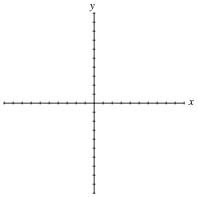On the same graph, plot the solutions to the equation y - x = 3. What is the significance of the point where the two lines cross?The point of intersection between the two lines represents the one solution set that satisfies both equations (where x = 2.5 and y = 5.5).

Notes:
The purpose of this question is to gently introduce students to the concept of simultaneous systems of equations, where a set of solutions satisfies more than one equation at a time. It is important for students to understand the basic concepts of graphing before they try to answer this question, though.

### Question 3:

What does it actually mean to obtain a solution for a ßimultaneous" system of equations? For example, if 2x + y = 7 and x - y = -1, what do the solution values (x = 2 ; y = 3) represent?
If we were to graph both these linear equations on a Cartesian (x, y) coordinate system, where would the solution (2,3) be located on the graph?
The solutions for a system of equations represent a unique combination of values that satisfy all equations in that system. For a two variable system, the solution is the intersection of two lines.

Notes:
Many students have difficulty grasping the significance of systems of equations. Discuss the meaning of equations, and systems of equations, with your students, making sure that the concept of simultaneity (solutions satisfying all equations at once) is made clear.

### Question 4:

Plot the equation y = x2 on the following graph: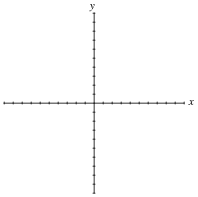On the same graph, plot the equation y = x + 2. What is the significance of the point where the two plots cross?Here there are two points of intersection between the parabola (curve) and the straight line, representing two different solution sets that satisfy both equations.
Challenge question: solve this simultaneous system of equations without graphing, but by symbolically manipulating the equations!

Notes:
Here, solution by graphing may be a bit easier than the symbolic solution. In principle we may determine solutions for any pair of equations by graphing, with about equal difficulty. The only real problem is precision: how closely we may interpret to points of intersection. A practical example of non-linear simultaneous function solution is load line analysis in semiconductor circuitry.

### Question 5:

Load lines are useful tools for analyzing transistor amplifier circuits, but they may be hard to understand at first. To help you understand what "load lines" are useful for and how they are determined, I will apply one to this simple two-resistor circuit: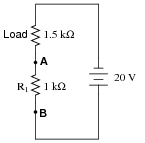We will have to plot a load line for this simple two-resistor circuit along with the "characteristic curve" for resistor R1 in order to see the benefit of a load line. Load lines really only have meaning when superimposed with other plots. First, the characteristic curve for R1, defined as the voltage/current relationship between terminals A and B: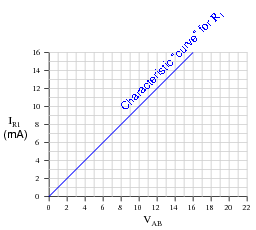Next, I will plot the load line as defined by the 1.5 kW load resistor. This "load line" expresses the voltage available between the same two terminals (VAB) as a function of the load current, to account for voltage dropped across the load: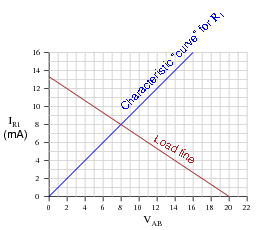At what value of current (IR1) do the two lines intersect? Explain what is significant about this value of current.
IR = 8 mA is the same value of current you would calculate if you had analyzed this circuit as a simple series resistor network.
Follow-up question: you might be wondering, "what is the point of plotting a 'characteristic curve' and a 'load line' in such a simple circuit, if all we had to do to solve for current was add the two resistances and divide that total resistance value into the total voltage?" Well, to be honest, there is no point in analyzing such a simple circuit in this manner, except to illustrate how load lines work. My follow-up question to you is this: where would plotting a load line actually be helpful in analyzing circuit behavior? Can you think of any modifications to this two-resistor circuit that would require load line analysis in order to solve for current?

Notes:
While this approach to circuit analysis may seem silly - using load lines to calculate the current in a two-resistor circuit - it demonstrates the principle of load lines in a context that should be obvious to students at this point in their study. Discuss with your students how the two lines are obtained (one for resistor R1 and the other plotting the voltage available to R1 based on the total source voltage and the load resistor's value).
Also, discuss the significance of the two line intersecting. Mathematically, what does the intersection of two graphs mean? What do the coordinate values of the intersection point represent in a system of simultaneous functions? How does this principle relate to an electronic circuit?

### Question 6:

Load lines are useful tools for analyzing transistor amplifier circuits, but they may be applied to other types of circuits as well. Take for instance this diode-resistor circuit: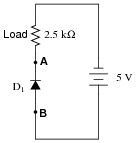The diode's characteristic curve is already plotted on the following graph. Your task is to plot the load line for the circuit on the same graph, and note where the two lines intersect: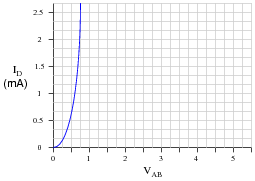What is the practical significance of these two plots' intersection?
The two lines intersect at a current of approximately 1.72 mA: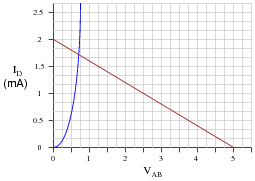Follow-up question: explain why the use of a load line greatly simplifies the determination of circuit current in such a diode-resistor circuit.
Challenge question: suppose the resistor value were increased from 2.5 kW to 10 kW. What difference would this make in the load line plot, and in the intersection point between the two plots?

Notes:
While this approach to circuit analysis may seem silly - using load lines to calculate the current in a diode-resistor circuit - it demonstrates the principle of load lines in a context that should be obvious to students at this point in their study. Discuss with your students how the load line is obtained for this circuit, and why it is straight while the diode's characteristic curve is not.
Also, discuss the significance of the two line intersecting. Mathematically, what does the intersection of two graphs mean? What do the coordinate values of the intersection point represent in a system of simultaneous functions? How does this principle relate to an electronic circuit?

### Question 7:

Suppose you were given the following two equations and asked to find solutions for x and y that will satisfy both at the same time:

 y + x = 8

 y - x = 3

If we manipulate the second equation so as to solve for y, we will have a definition of y in terms of x that we may use for substitution in the first equation:

 y = x + 3

Show the process of substitution into the first equation, and how this leads to a single solution for x. Then, use that value of x to solve for y, resulting in a solution set valid for both equations.
If y + x = 8 and y = x + 3, then (x + 3) + x = 8. Therefore,

 x = 2.5 and y = 5.5

Notes:
This question demonstrates one of the (many) practical uses of algebraic substitution: solving simultaneous systems of equations.

### Question 8:

An interesting and useful property in mathematics is the transitive property:

 If a = b and b = c , then a = c

Simply stated, two variables must be equal to one another if they are both equal to a common (third) variable. While not particularly profound or breathtaking in scope, this property is nevertheless useful in solving certain mathematical problems.
Suppose you were given the following two equations and asked to find solutions for x and y that will satisfy both at the same time:

 y + x = 8

 y - x = 3

Manipulate both of these equations to solve for y, and then explain how you could apply the transitive principle to solve for x.
If 8 - x = y and 3 + x = y, then 8 - x must equal 3 + x:

 8 - x = 3 + x

Solutions for x and y:

 x = 2.5 and y = 5.5

Notes:
This method of solving for a two-variable set of simultaneous equations is really nothing more than substitution in disguise. Some students find it easier to grasp than straight substitution, though.

### Question 9:

Suppose you were given the following two equations and asked to find solutions for x and y that will satisfy both at the same time:

 y + x = 8

 y - x = 3

Now, you know that we may do anything we want to either equation as long as we do the same thing to both sides (on either side of the ëqual" sign). This is the basic rule we follow when manipulating an equation to solve for a particular variable. For example, we may take the equation y + x = 8 and subtract x from both sides to yield an equation expressed in terms of y: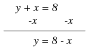Following the same principle, we may take two equations and combine them either by adding or subtracting both sides. For example, we may take the equation y - x = 3 and add both sides of it to the respective sides of the first equation y + x = 8: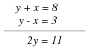What beneficial result comes of this action? In other words, how can I use this new equation 2y = 11 to solve for values of x and y that satisfy both of the original equations?
We may use the result (2y = 11) to solve for a value of y, which when substituted into either of the original equations may be used to solve for a value of x to satisfy both equations at the same time.

Notes:
While not intuitively obvious to most people, the technique of adding two entire equations to each other for the purpose of eliminating a variable is not only possible to do, but very powerful when looking for solutions to satisfy both original equations. Discuss with your students why it is allowable for us to add y - x to y + x and to add 3 to 8. to yield the equation 2y = 11.

### Question 10:

Solve for values of x and y that will satisfy both of the following equations at the same time:

 x + 2y = 9

 4x - y = -18

x = -3          y = 6
Follow-up question: solve this system of simultaneous equations using both substitution (solving for one variable in one of the equations and substituting that into the other equation) and addition (adding the two equations together to produce a third equation with only one unknown).

Notes:
Nothing special here - just practice solving for a two-variable system of equations.

### Question 11:

Solve for values of x and y that will satisfy both of the following equations at the same time:

 3x - y = 17

 x + 2y = 1

x = 5               y = -2

Notes:
Nothing but "drill" (practice) here.

### Question 12:

Solve for values of x and y that will satisfy both of the following equations at the same time:

 3x - y = -9

 x + 2y = 4

x = -2               y = 3

Notes:
Nothing but "drill" (practice) here.

### Question 13:

If we wish to solve for the value of three inter-related variables (i.e. x + y + z = 0), how many equations do we need in our ßystem" of simultaneous equations, total?
Graphically, what does the solution set (x,y,z) represent for a system of equations with three variables?
Three variables require three equations for solution. Graphically, the solution set represents the point at which three planes of infinite area intersect.

Notes:
Ask your students to graphically contrast the scenario of three variables and three equations against two variables and two equations. Where is the solution set represented in a two-variable, two-equation system? How many we extrapolate from this situation to one where three variables and three equations are involved?

### Question 14:

Many circuit analysis techniques require the solution of ßystems of linear equations," sometimes called ßimultaneous equations." This question is really a series of practice problems for solving simultaneous linear equations, the purpose being to give you lots of practice using various solution techniques (including the solution facilities of your calculator).
Systems of two variables:
 x + y = 5 x - y = -6 2x + y = 7

 x - y = 1 2x - y = 4 x - y = 2

 3x - 2y = -1 -10x + 2y = 0 3x - 5y = -13

 5x + y = -6 -3x - 5y = -28 -x + 2y = 5

 1000x - 500y = 0 -15000x + 2200y = -66200 9100x - 5000y = 24

 550x + 2500y = 5550 7900x - 2800y = 28300 -5200x - 2700y = -6.5

Systems of three variables:
 x - y + z = 1 3x + 2y - 5z = -21 x + y + z = 0

 -x - y + z = -1 x - 3y + z = 8 2x - y - 4z = -9

 x + y + z = 3 -x - y - z = -12 -2x + 2y - z = 12

 x + y - 2z = -12 -4x - 3y + 2z = -32 19x - 6y + 20z = -33

 3x - 2y + z = 19 x - 2y + 3z = -1 4x + 5y - 3z = -17

 -4x + 3y - 5z = -45 -2x + 7y - z = 3 -7x + 2y - 8z = 9

 890x - 1000y + 2500z = -1500 2750x - 6200y + 4500z = 17500

 3300x + 7200y - 5100z = 21500 -10000x + 5300y - 1000z = 8100

 -x + y - z = 0 6x - 2y - 3z = 5

Systems of two variables:
 x + y = 5 x - y = -6 2x + y = 7

 x - y = 1 2x - y = 4 x - y = 2

 x = 3 ; y = 2 x = 10 ; y = 16 x = 3 ; y = 1

 3x - 2y = -1 -10x + 2y = 0 3x - 5y = -13

 5x + y = -6 -3x - 5y = -28 -x + 2y = 5

 x = -1 ; y = -1 x = 1 ; y = 5 x = -1 ; y = 2

 1000x - 500y = 0 -15000x + 2200y = -66200 9100x - 5000y = 24

 550x + 2500y = 5550 7900x - 2800y = 28300 -5200x - 2700y = -6.5

 x = 1 ; y = 2 x = 5 ; y = 4 x = 0.001924 ; y = -0.001298

Systems of three variables:
 x - y + z = 1 3x + 2y - 5z = -21 x + y + z = 0

 -x - y + z = -1 x - 3y + z = 8 2x - y - 4z = -9

 x + y + z = 3 -x - y - z = -12 -2x + 2y - z = 12

 x = 1 ; y = 1 ; z = 1 x = 4 ; y = 1 ; z = 7 x = -3 ; y = 3 ; z = 0

 x + y - 2z = -12 -4x - 3y + 2z = -32 19x - 6y + 20z = -33

 3x - 2y + z = 19 x - 2y + 3z = -1 4x + 5y - 3z = -17

 -4x + 3y - 5z = -45 -2x + 7y - z = 3 -7x + 2y - 8z = 9

 x = 2 ; y = -4 ; z = 5 x = 6 ; y = 2 ; z = -1 x = -5 ; y = 3 ; z = 4

 890x - 1000y + 2500z = -1500 2750x - 6200y + 4500z = 17500

 3300x + 7200y - 5100z = 21500 -10000x + 5300y - 1000z = 8100

 -x + y - z = 0 6x - 2y - 3z = 5

 x = 2.215 ; y = 1.378 ; z = -0.8376 x = -5.171 ; y = -9.322 ; z = -5.794

Notes:
I suggest you let your students discover how to use the equation-solving facilities of their scientific calculators on their own. My experience has been that students both young and old take to this challenge readily, because they realize learning how to use their calculators will save them a tremendous amount of hand calculations!

### Question 15:

Suppose you needed to choose a fixed resistor value (R) to make a voltage divider circuit, given a known potentiometer resistance value, the source voltage value, and the desired range of adjustment: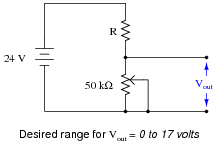Solve for R, and show the equation you set up in order to do it.
Hint: remember the series resistor voltage divider formula . . .

 VR = Vtotal �� R Rtotal ��

R = 20.588 kW

Notes:
Be sure to have your students set up their equations in front of the class so everyone can see how they did it. Some students may opt to apply Ohm's Law to the solution of R, which is good, but for the purpose of developing equations to fit problems it might not be the best solution. Challenge your students to come up with a single equation that solves for R, with all known quantities on the other side of the ëqual" sign.

### Question 16:

Suppose you needed to choose a potentiometer value (R) to make a voltage divider circuit, given a known fixed resistor value, the source voltage value, and the desired range of adjustment: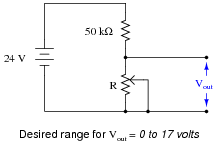Solve for R, and show the equation you set up in order to do it.
Hint: remember the series resistor voltage divider formula . . .

 VR = Vtotal �� R Rtotal ��

R = 121.43 kW
Follow-up question: you will not be able to find a potentiometer with a full-range resistance value of exactly 121.43 kW. Describe how you could take a standard-value potentiometer and connect it to one or more fixed-value resistors to give it this desired full-scale range.

Notes:
Be sure to have your students set up their equations in front of the class so everyone can see how they did it. Some students may opt to apply Ohm's Law to the solution of R, which is good, but for the purpose of developing equations to fit problems it might not be the best solution. Challenge your students to come up with a single equation that solves for R, with all known quantities on the other side of the ëqual" sign.
The follow-up question is very practical, as it is impossible to find potentiometers ready-made to arbitrary values of full-scale resistance. Instead, you must work with what you can find, which is usually nominal values such as 10 kW, 100 kW, 1 MW, etc.

### Question 17:

An engineer needs to calculate the values of two resistors to set the minimum and maximum resistance ratios for the following potentiometer circuit: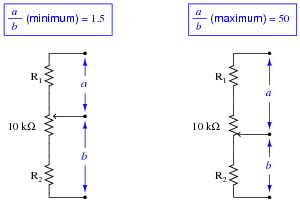First, write an equation for each circuit, showing how resistances R1, R2, and the 10 kW of the potentiometer combine to form the ratio [a/b]. Then, use techniques for solving simultaneous equations to calculate actual resistance values for R1 and R2.

 a b (minimum) = R1 R2 + 10000 a b (maximum) = R1 + 10000 R2

R1 = 15.77 kW
R2 = 515.5 W

Notes:
This very practical application of simultaneous equations was actually used by one of my students in establishing the lower and upper bounds for the voltage gain adjustment of an inverting opamp circuit!

### Question 18:

The voltage gain of a common-emitter transistor amplifier is approximately equal to the collector resistance divided by the emitter resistance: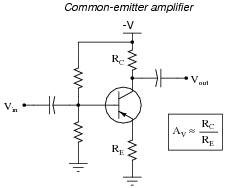Knowing this, calculate the necessary resistance values for the following fixed-value resistor (R2) and potentiometer (R1) to give this common-emitter amplifier an adjustable voltage gain range of 2 to 8: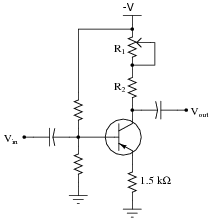R1 (pot) = 9 kW
R2 (fixed) = 3 kW

Notes:
Ask your students how they might make a standard-value potentiometer such as 10 kW have a full-scale (maximum) resistance of only 9 kW.

### Question 19:

The voltage gain of a common-emitter transistor amplifier is approximately equal to the collector resistance divided by the emitter resistance: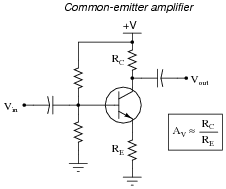Knowing this, calculate the necessary resistance values for the following fixed-value resistors (R1 and R2) to give this common-emitter amplifier an adjustable voltage gain range of 4 to 7: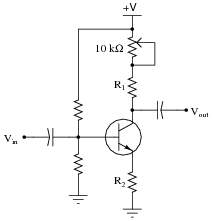R1 = 13.33 kW
R2 = 3.333 kW

Notes:
Have your students show how they set up the system of equations to solve for the two resistor values. This is a good exercise to do in front of the class, so everyone can see (possibly) different methods of solution.

### Question 20:

Suppose you needed to choose two resistance values to make a voltage divider with a limited adjustment range. One of these resistors will be fixed in value (R1), while the other will be variable (a potentiometer connected as a rheostat- R2):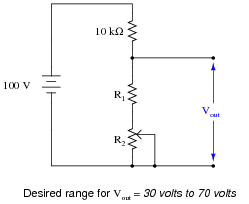Set up a system of simultaneous equations to solve for both R1 and R2, and show how you arrived at the solutions for each.
Hint: remember the series resistor voltage divider formula . . .

 VR = Vtotal �� R Rtotal ��

R1 (fixed) = 4.286 kW
R2 (pot) = 19.048 kW
Follow-up question: you will not be able to find a potentiometer with a full-range resistance value of exactly 19.048 kW. Describe how you could take a standard-value potentiometer and connect it to one or more fixed-value resistors to give it this desired full-scale range.

Notes:
Be sure to have your students set up their equations in front of the class so everyone can see how they did it. Some students may opt to apply Ohm's Law to the solution of both resistors, which is good, but for the purpose of developing equations to fit problems it might not be the best solution. Challenge your students to come up with a set of equations that solve for R1 and R2, then use techniques for solution of simultaneous equations to arrive at solutions for each.
The follow-up question is very practical, as it is impossible to find potentiometers ready-made to arbitrary values of full-scale resistance. Instead, you must work with what you can find, which is usually nominal values such as 10 kW, 50 kW, 100 kW, etc.

### Question 21:

Suppose you needed to choose two resistance values to make a voltage divider with a limited adjustment range:Set up a system of simultaneous equations to solve for both R1 and R2, and show how you arrived at the solutions for each.
Hint: remember the series resistor voltage divider formula . . .

 VR = Vtotal �� R Rtotal ��

R1 = 5.25 kW
R2 = 2.25 kW

Notes:
Be sure to have your students set up their equations in front of the class so everyone can see how they did it. Some students may opt to apply Ohm's Law to the solution of both resistors, which is good, but for the purpose of developing equations to fit problems it might not be the best solution. Challenge your students to come up with a set of equations that solve for R1 and R2, then use techniques for solution of simultaneous equations to arrive at solutions for each.

### Question 22:

Use simultaneous equations to calculate the values of R1 and R2 necessary to give this voltage divider the range of adjustment specified:Vout (minimum) = 3 volts          Vout (maximum) = 8 volts

R1 =                        R2 =
R1 = 2 kW
R2 = 3 kW

Notes:
Have your students show their methods of solution in class, so you may observe their problem-solving ability and they may see multiple methods of solution.

### Question 23:

Use simultaneous equations to calculate the values of R1 and R2 necessary to give this voltage divider the range of adjustment specified: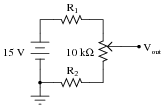Vout (minimum) = 5 volts          Vout (maximum) = 12 volts

R1 =                        R2 =
R1 = 4.2857 kW
R2 = 7.1429 kW

Notes:
Have your students show their methods of solution in class, so you may observe their problem-solving ability and they may see multiple methods of solution.

### Question 24:

The voltage gain of an inverting operational amplifier circuit is defined by the ratio of feedback to input resistance:

 AV = Rf Ri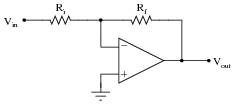Calculate the necessary values of R1 and R2 to limit the minimum and maximum voltage gain of this opamp circuit to 5 and 30, respectively, given a potentiometer in the middle with a full-span resistance of 5 kW: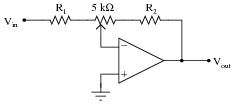R1 = 1.2 kW
R2 = 31 kW

Notes:
This is a very practical example of using simultaneous equations in analog circuit design.

### Question 25:

Calculate the necessary values of R1 and R2 to limit the minimum and maximum voltage gain of this opamp circuit to 10 and 85, respectively: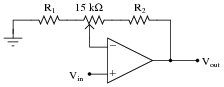R1 = 2 kW
R2 = 153 kW

Notes:
This is a very practical example of using simultaneous equations in analog circuit design. A common mistake students make while setting up the equations is forgetting that a noninverting amplifier's gain is the ratio of the feedback and grounding resistors, plus one!